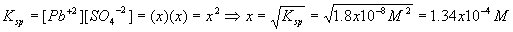Chemical Equilibrium – Solubility Products

Solubility Product

Now we consider some problems in solution equilibrium. There are two broad cases to be considered - very soluble salts such as NaCl which dissociate 100% upon addition to waterand insoluble salts which barely dissolve and always have solid precipitate present. A typical example isThis describes lead (Pb+2) and sulfate (SO4-2) ions dissolved in solution, the aqueous phase (aq), in equilibrium with the sparingly soluble solid (s) salt PbSO4. Similarly to the other chemical equilibrium problems we have seen, there is an equilibrium constant, Ksp, defined asAs long as solid PbSO4 is present, the solution is not only at equilibrium, but is also saturated (contains as much dissolved salt as it can hold).

Example:

Solid PbSO4 is placed in water and the resulting mixture is left alone for a long time. There is still solid remaining after the waiting time, and the solution is presumed to be in equilibrium. What are the equilibrium concentrations of the two ionic species,

Pb+2 and SO4-2 ? The value of Ksp is 1.8x10-8 M2.

Solution:

Write a start-change-finish diagram, assuming the amount of solid lead sulfate is infinite

(subtracting the small amount that dissolves doesn’t matter):

 Component Pb+2 SO4-2 PbSO4 Start 0 0 N/A Change + x + x - x Finish x x N/A

Plugging in to the equilibrium equation gives usSo

[Pb+2] = [SO4-2] = x = 1.34x10-4 M.

As you can see, these types of problems are usually much easier to solve algebraically than the chemical equilibrium problems considered earlier.

Example

Solid Cadmium Arsenate, Cd3(AsO4)2 , is in equilibrium with its own saturated solution. The salt has a solubility product constant Ksp = 2.2x10-32. What are the concentrations of the two ionic species Cd+2 and AsO4-3 ?

Solution

Notice that the stoichiometric coefficients are important, since they are not all one as in the previous example, so write a chemical reaction firstNow, write a start-change-finish diagram with the same assumptions as before, but notice how the stoichiometric coefficients change the diagram

 Component Cd3(AsO4)2 Cd+2 AsO4-3 Start N/A 0 0 Change N/A + 3x + 2x Finish N/A 3x 2xTherefore,

[Cd+2] = 3x = 3(1.8x10-7) = 5.5x10-7 M

[AsO4-3] = 2x = 2(1.8x10-7) = 3.7 x10-7 M

Soluble or insoluble?

There are rules to help decide whether a compound is likely to be soluble or insoluble in water. These are almost always listed in any good freshman chemistry text. Here's a drastically simplified rule: The farther apart the chemical species are on the periodic table, the more likely it is that the salt is soluble. This rule is not very helpful when talking about compound ions like NH4+ (all compounds soluble, by the way) or SO3-2

(depends on the cation whether soluble or not), but does provide some intuition for simple salts such as NaBr (soluble), Cu2S (insoluble) and AuI (insoluble).